# WarmUp Slope Objectives Define slope as the ratio

• Slides: 14Warm-Up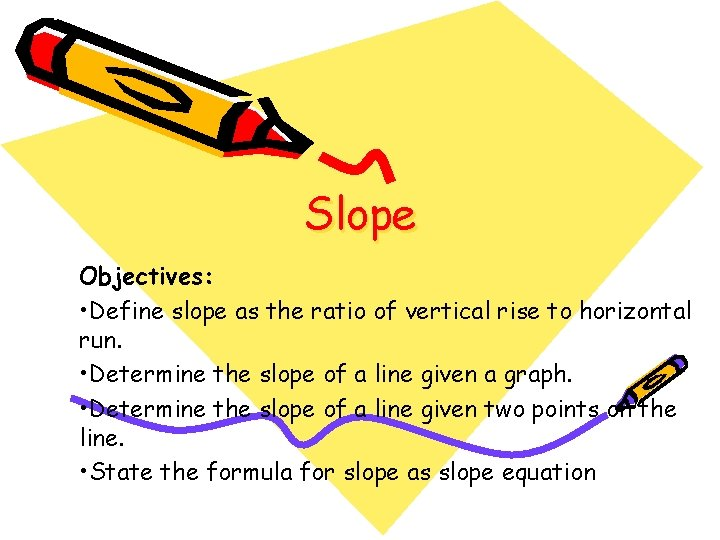Slope Objectives: • Define slope as the ratio of vertical rise to horizontal run. • Determine the slope of a line given a graph. • Determine the slope of a line given two points on the line. • State the formula for slope as slope equationSlope 10 run 8 Slope = 6 4 rise 2 -4 -2 -2 -4 -6 -8 -10 2 4 6 8 10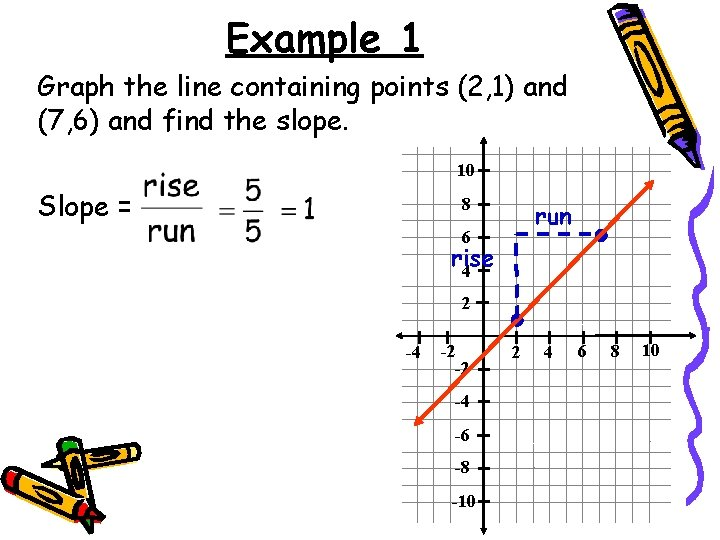Example 1 Graph the line containing points (2, 1) and (7, 6) and find the slope. 10 Slope = 8 run 6 rise 4 2 -4 -2 -2 -4 -6 -8 -10 2 4 6 8 10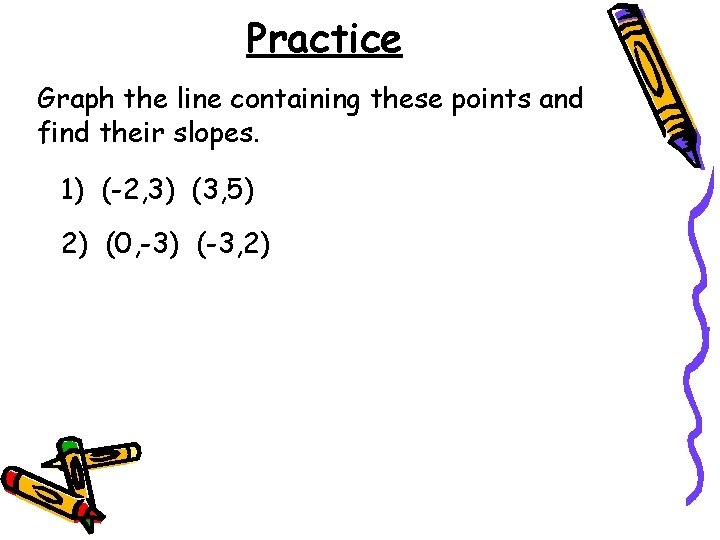Practice Graph the line containing these points and find their slopes. 1) (-2, 3) (3, 5) 2) (0, -3) (-3, 2)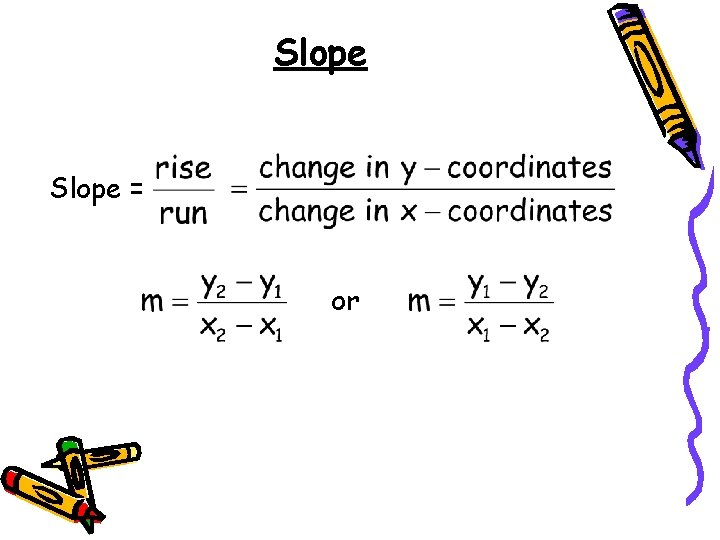Slope = orExample 2 Find the slope of the line containing points (1, 6) and (5, 4).Practice Find the slope of the lines containing these points. 1) (2, 2) (8, 9) 3) (5, -11) (-9, 4) 2) (-2, 3) (2, 1)Slope of a Horizontal Line What about the slope of a horizontal line? What is the rise? 0 10 8 What is the run? 6 6 4 Slope = The slope of ANY horizontal line is 0. 2 -4 -2 -2 -4 -6 -8 -10 2 4 6 8 10Slope of a Vertical Line What about the slope of a vertical line? What is the rise? 5 10 8 What is the run? 0 6 4 Slope = The slope of ANY vertical line is undefined. 2 -4 -2 -2 -4 -6 -8 -10 2 4 6 8 10Example 1 Find the slope of the line y = -4. 10 8 6 Slope = 4 2 -4 -2 -2 -4 -6 -8 -10 2 4 6 8 10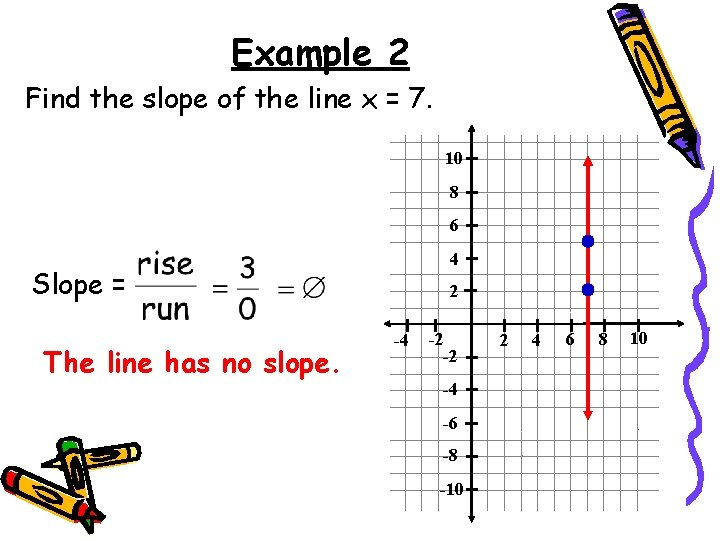Example 2 Find the slope of the line x = 7. 10 8 6 4 Slope = The line has no slope. 2 -4 -2 -2 -4 -6 -8 -10 2 4 6 8 10Practice Find the slopes, if they exist, of the lines containing these points. 1) (9, 7) (3, 7) 3) (2, 4) (-1, 5) 2) (4, -6) (4, 0)Homework Worksheet on Slope